# Exterior algebra

Grassmann algebra, of a vector spaceover a fieldAn associative algebra over, the operation in which is denoted by the symbol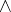, with generating elementswhere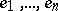is a basis of, and with defining relations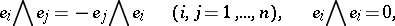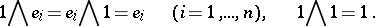The exterior algebra does not depend on the choice of the basis and is denoted by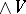. The subspace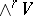() ingenerated by the elements of the form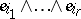is said to be the-th exterior power of the space. The following equalities are valid: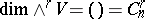,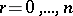,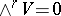,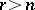. In addition,if,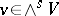. The elements of the spaceare said to be-vectors; they may also be regarded as skew-symmetric-times contravariant tensors in(cf. Exterior product).-vectors are closely connected with-dimensional subspaces in: Linearly independent systems of vectors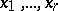andofgenerate the same subspace if and only if the-vectorsand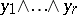are proportional. This fact served as one of the starting points in the studies of H. Grassmann , who introduced exterior algebras as the algebraic apparatus to describe the generation of multi-dimensional subspaces by one-dimensional subspaces. The theory of determinants is readily constructed with the aid of exterior algebras. An exterior algebra may also be defined for more general objects, viz. for unitary modulesover a commutative ringwith identity . The-th exterior power,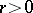, of a moduleis defined as the quotient module of the-th tensor power of this module by the submodule generated by the elements of the form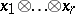, where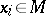and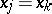for certain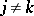. The exterior algebra foris defined as the direct sum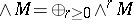, where, with the naturally introduced multiplication. In the case of a finite-dimensional vector space this definition and the original definition are identical. The exterior algebra of a module is employed in the theory of modules over a principal ideal ring .

The Grassmann (or Plücker) coordinates of an-dimensional subspacein an-dimensional spaceoverare defined as the coordinates of the-vector incorresponding to, which is defined up to proportionality. Grassmann coordinates may be used to naturally imbed the set of all-dimensional subspaces ininto the projective space of dimension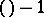, where it forms an algebraic variety (called the Grassmann manifold). Thus one gets several important examples of projective algebraic varieties .

Exterior algebras are employed in the calculus of exterior differential forms (cf. Differential form) as one of the basic formalisms in differential geometry , . Many important results in algebraic topology are formulated in terms of exterior algebras.

E.g., ifis a finite-dimensional-space (e.g. a Lie group), the cohomology algebra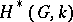ofwith coefficients in a fieldof characteristic zero is an exterior algebra with odd-degree generators. Ifis a simply-connected compact Lie group, then the ring, studied in-theory, is also an exterior algebra (over the ring of integers).